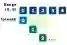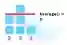# C# IEnumerable Examples

Use the IEnumerable interface. IEnumerable things can be looped over with foreach.

IEnumerable. One season comes after another. In winter a chill settles on the forest. All things freeze. In spring, warmth thaws the land and life begins again.On enumerating. With time, we enumerate seasons. IEnumerable things (like arrays or Lists) have elements that come one after another. We can enumerate elements in loops.Interface

Query example. Here we use IEnumerable as the return value of a query expression. We use it with foreach-loops, and also call extension methods on it.
Part 1: An IEnumerable is returned from a query expression. A query that selects ints will be of type IEnumerable<int>.
Part 2: On an IEnumerable variable, we can use the foreach-loop. This loop iterates with simple syntax.C# program that uses IEnumerable using System; using System.Collections.Generic; using System.Linq; class Program { static void Main() { // Part 1: query expression. IEnumerable<int> result = from value in Enumerable.Range(0, 5) select value; // Part 2: loop over IEnumerable. foreach (int value in result) { Console.WriteLine(\$"IENUMERABLE: {value}"); } } } Output IENUMERABLE: 0 IENUMERABLE: 1 IENUMERABLE: 2 IENUMERABLE: 3 IENUMERABLE: 4

Extension methods. In System.Linq, many extension methods that act upon IEnumerable are available. These are used in many C# programs.
Part A: Here we see the Average extension, which computes the average value in an IEnumerable (like an int array).
Part B: We can apply many transformations to an IEnumerable instance, including the ToList and ToArray conversions.C# program that uses IEnumerable extension methods using System; using System.Collections.Generic; using System.Linq; class Program { static void Main() { int[] values = new int[] { 2, 3, 1 }; // Part A: use Average extension method on IEnumerable. double average = values.Average(); Console.WriteLine(\$"AVERAGE: {average}"); // Part B: convert IEnumerable with ToList extension. List<int> list = values.ToList(); Console.WriteLine(\$"TOLIST: {list.Count}"); } } Output AVERAGE: 2 TOLIST: 3

Arguments. Many classes implement IEnumerable. We can pass them directly to methods that receive IEnumerable arguments. The type parameter must be the same.
Next: Display() receives an IEnumerable argument. We can pass Lists or arrays to it.
Also: We can implement IEnumerable on a type to provide support for the foreach-loop. This is done through the GetEnumerator method.
C# program that uses IEnumerable argument using System; using System.Collections.Generic; class Program { static void Main() { Display(new List<bool> { true, false, true }); } static void Display(IEnumerable<bool> argument) { foreach (bool value in argument) { Console.WriteLine(value); } } } Output True False True

2D array. IEnumerable works with a 2D array. It enables a foreach-loop over the values in a 2D or jagged array. We create a custom method that returns IEnumerable.2D Array
Foreach: We use IEnumerable and the foreach-loop to access, in sequence, all items in a 2D array. We can abstract the loop itself out.
Here: This example shows the yield contextual keyword in a method that returns IEnumerable<T>.
Generic: The return value is a generic IEnumerable collection of ints. We must specify the int in angle brackets.
Generic Class, MethodInt, uint
C# program that uses array, yield keyword using System; using System.Collections.Generic; class Program { static int[,] _grid = new int[15, 15]; static void Main() { // Initialize some elements in 2D array. _grid[0, 1] = 4; _grid[4, 4] = 5; _grid[14, 2] = 3; // Sum values in 2D array. int sum = 0; foreach (int value in GridValues()) { sum += value; } // Write result. Console.WriteLine("SUMMED 2D ELEMENTS: " + sum); } public static IEnumerable<int> GridValues() { // Use yield return to return all 2D array elements. for (int x = 0; x < 15; x++) { for (int y = 0; y < 15; y++) { yield return _grid[x, y]; } } } } Output SUMMED 2D ELEMENTS: 12

GetEnumerator error. In a class, the foreach-loop is not by default supported. A GetEnumerator method (often part of the IEnumerable interface) is required.
So: We can implement IEnumerable to fix this error on a class. This provides the GetEnumerator method.
C# program that causes GetEnumerator error using System; class Example { } class Program { static void Main() { Example example = new Example(); // This does not compile: GetEnumerator is required. foreach (string element in example) { Console.WriteLine(true); } } } Output error CS1579: foreach statement cannot operate on variables of type 'Example' because 'Example' does not contain a public definition for 'GetEnumerator'

Implement IEnumerable. This example implements the IEnumerable interface on an Example class. The class contains a List, and for GetEnumerator, we use the List's GetEnumerator method.
So: We forward the details of the implementation to the List. Our IEnumerable implementation relies on another.
Main: Here we call the Example class constructor, which populates the _elements field.
Foreach: The foreach-loop in the Main method implicitly (secretly) calls the GetEnumerator method. So "HERE" is written.
Result: The program compiles. The foreach loop refers to the List's Enumerator and loops over the elements of the List.
C# program that implements IEnumerable using System; using System.Collections; using System.Collections.Generic; class Example : IEnumerable<string> { List<string> _elements; public Example(string[] array) { this._elements = new List<string>(array); } IEnumerator<string> IEnumerable<string>.GetEnumerator() { Console.WriteLine("HERE"); return this._elements.GetEnumerator(); } IEnumerator IEnumerable.GetEnumerator() { return this._elements.GetEnumerator(); } } class Program { static void Main() { Example example = new Example( new string[] { "cat", "dog", "bird" }); // The foreach-loop calls the generic GetEnumerator method. // ... It then uses the List's Enumerator. foreach (string element in example) { Console.WriteLine(element); } } } Output HERE cat dog bird

Range. Some methods, like Enumerable.Range(), make it easier to create IEnumerable collections. We do not need to create a separate array.
Program: We test Enumerable.Range, which returns an IEnumerable collection. We enumerate its return value with foreach.
Range, Repeat, Empty
Info: Other methods, like Enumerable.Repeat and Empty, are available. They can be useful in certain programs.
C# program that uses Enumerable.Range using System; using System.Linq; class Program { static void Main() { // Get IEnumerable from Enumerable.Range and loop over it. foreach (int value in Enumerable.Range(100, 2)) { Console.WriteLine("RANGE(0, 2): {0}", value); } } } Output RANGE(0, 2): 100 RANGE(0, 2): 101

Benchmark, IEnumerable. A single IEnumerable method can reduce code size—this has speed advantages. For numeric methods, though, using an array directly is usually a faster approach.
Version 1: We loop over the 3 elements in an int array with for each, and sum them. No IEnumerable is involved.
Version 2: We use AsEnumerable to get an IEnumerable of the int array. Then we use foreach over the IEnumerable.
AsEnumerable
Result: The IEnumerable foreach-loop is much slower. It is best to access arrays directly for most numeric operations.
C# program that tests IEnumerable performance using System; using System.Diagnostics; using System.Linq; class Program { const int _max = 1000000; static void Main() { int[] values = { 10, 20, 30 }; // Version 1: loop over array directly. var s1 = Stopwatch.StartNew(); for (int i = 0; i < _max; i++) { int sum = 0; foreach (int value in values) { sum += value; } if (sum == 0) { return; } } s1.Stop(); // Version 2: convert array to IEnumerable and loop over IEnumerable elements. var s2 = Stopwatch.StartNew(); for (int i = 0; i < _max; i++) { int sum = 0; var enumerable = values.AsEnumerable(); foreach (int value in enumerable) { sum += value; } if (sum == 0) { return; } } s2.Stop(); Console.WriteLine(((double)(s1.Elapsed.TotalMilliseconds * 1000000) / _max).ToString("0.00 ns")); Console.WriteLine(((double)(s2.Elapsed.TotalMilliseconds * 1000000) / _max).ToString("0.00 ns")); } } Output 1.96 ns int[], foreach 25.77 ns IEnumerable int, foreach

Implement, shortcut. In Visual Studio we can implement an interface with some help. Hover the mouse over the IEnumerable name after specifying it.
Then: Select from the menu one of the options. The first is probably the best choice.

Yield. This keyword is placed before "return." It is used in methods that return IEnumerable. We can use yield to "save the state" of the function after the return.Yield

Foreach. This kind of loop has advantages. It results in simpler, clearer syntax. We no longer need to track indexes with variables (which often have names like i or x).Foreach

Safer, more robust. With IEnumerable, our code becomes safer and more robust. It does this by hiding all the details about the underlying collection.

Extensions. Consider this. If a method needs to be implemented for every kind of List and Array (such as string lists, string arrays, int lists), our program will grow.
But: But if a single extension method, one receiving IEnumerable, can be implemented, our code base remains smaller.
Tip: A single extension can replace many specific methods. This can help keep programs small and easy to maintain.
Extension

IOrderedEnumerable. If we use an orderby clause in a query expression, we receive an IOrderedEnumerable. This can be used in the same way as an IEnumerable—we can loop over it.IOrderedEnumerable

A summary. This generic interface provides an abstraction for looping over elements. It provides foreach-loop support. And it allows us to use LINQ extensions.

Home

© 2007-2020 sam allen. send bug reports to info@dotnetperls.com.